# Java String startsWith(): Complete Guide

Java String startsWith() method is used to check the prefix of String. It verifies if the given String starts with an argument string or not. Java String startsWith() method is an overloaded method and has two forms:

1. public boolean startsWith(String prefix)
2. public boolean startsWith(String prefix, int index)

## Java String startsWith()

Java string startsWith() is a built-in method of String class used to check the prefix of the String. The startsWith() method can either start checking from the first index or check from a specified index. It returns the boolean value true or false based on whether the given String begins with the specified letter or word.

At times it is required to check whether a particular string starts with a given prefix or not. Java takes care of this through its java.lang.String.startsWith() method.

This leads to two variants of the method:

### boolean startsWith(String str):

It returns true if the str is a prefix of the String.

### boolean startsWith(String str, int fromIndex):

It returns true if the String begins with str starting from the specified index fromIndex. For example, let’s say that the value of a String s is “Hi there,” and we are calling starsWith() method like this: s.startsWith(“there”, 3) then this will return true because we have passed value 3 as fromIndex, the searching of the keyword “there” begins from an index 3 of the given string s. So it is found at the beginning of a string s.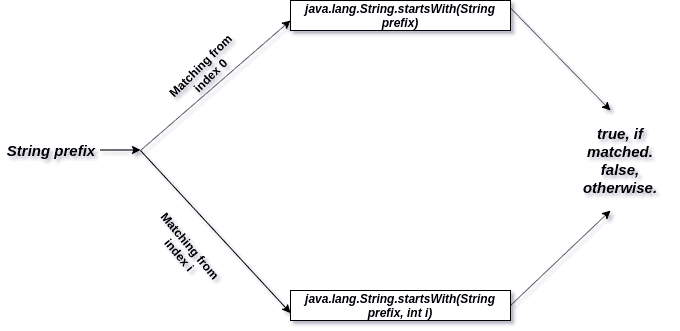## Internal Implementation

```public boolean startsWith(String prefix, int toffset) {
char ta[] = value;
int to = toffset;
char pa[] = prefix.value;
int po = 0;
int pc = prefix.value.length;
// Note: toffset might be near -1>>>1.
if ((toffset < 0) || (toffset > value.length - pc)) {
return false;
}
while (--pc >= 0) {
if (ta[to++] != pa[po++]) {
return false;
}
}
return true;
}```

## Starting at the first index

### Syntax

public boolean startsWith(String prefix)

### Parameters

The String that needs to be found at the beginning.

### Return value

It returns True if the match is found; otherwise, it returns False.

#### Example1.java:

The following example demonstrates the use of the startswith() method beginning the match from the first index.

See the following code.

```public class Example1 {
public static void main(String[] args) {
String s1 = new String("This is Java.");
System.out.println(s1);

System.out.println(s1.startsWith("This"));
}
}
```

#### Output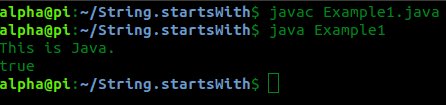#### Example2.java:

The following example demonstrates a similar situation.

```public class Example2 {
public static void main(String[] args) {
String s1 = new String("This is Java.");
System.out.println(s1);

System.out.println(s1.startsWith("Th"));
}
}
```

#### Output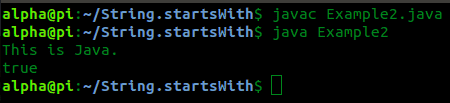#### Example3.java:

The following example demonstrates a failed match.

```public class Example3 {
public static void main(String[] args) {
String s1 = new String("This is Java.");
System.out.println(s1);

System.out.println(s1.startsWith("Java"));
}
}
```

#### Output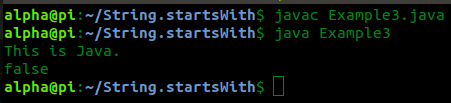## Starting at any given index

### Syntax

public boolean startsWith(String prefix, int index)

### Parameters

The String that needs to be found and the index from which to start matching from

### Return value

It returns true if a match is found, otherwise false.

#### Example4.java:

The following example demonstrates the use of the startsWith() function to start from any given index:

See the following program.

```public class Example4 {
public static void main(String[] args) {
String s1 = new String("This is Java.");
System.out.println(s1);
System.out.println(s1.startsWith("This", 8));
}
}
```

#### Output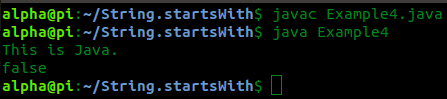#### Example5.java:

The following example demonstrates a failed match in this variation:

```public class Example5 {
public static void main(String[] args) {
String s1 = new String("This is Java.");
System.out.println(s1);

System.out.println(s1.startsWith("Java", 8));
}
}
```

#### Output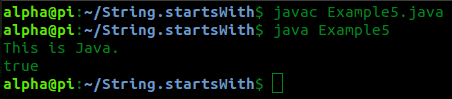This is how the java.lang.String.startsWith() method can match a string with a given prefix either starting from the first index or any given index.

## Conclusion

The method startsWith() is a convenience method that checks whether a string starts with another String. We can also pass the index of the first character to start checking from.

That’s it for this tutorial.

## Related Posts

Java String split()

Java String join()

Java String intern()

Java String replace()

Java String replaceAll()

This site uses Akismet to reduce spam. Learn how your comment data is processed.